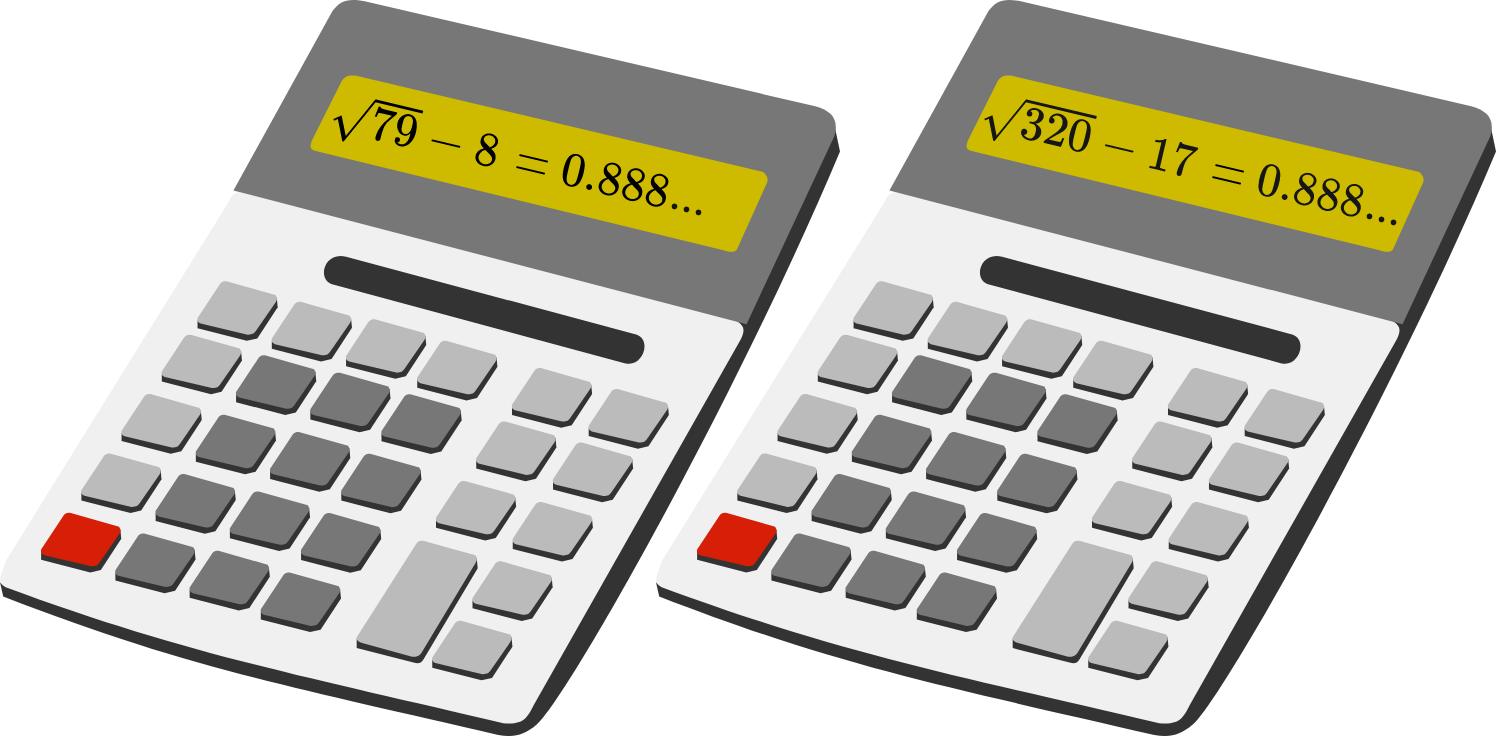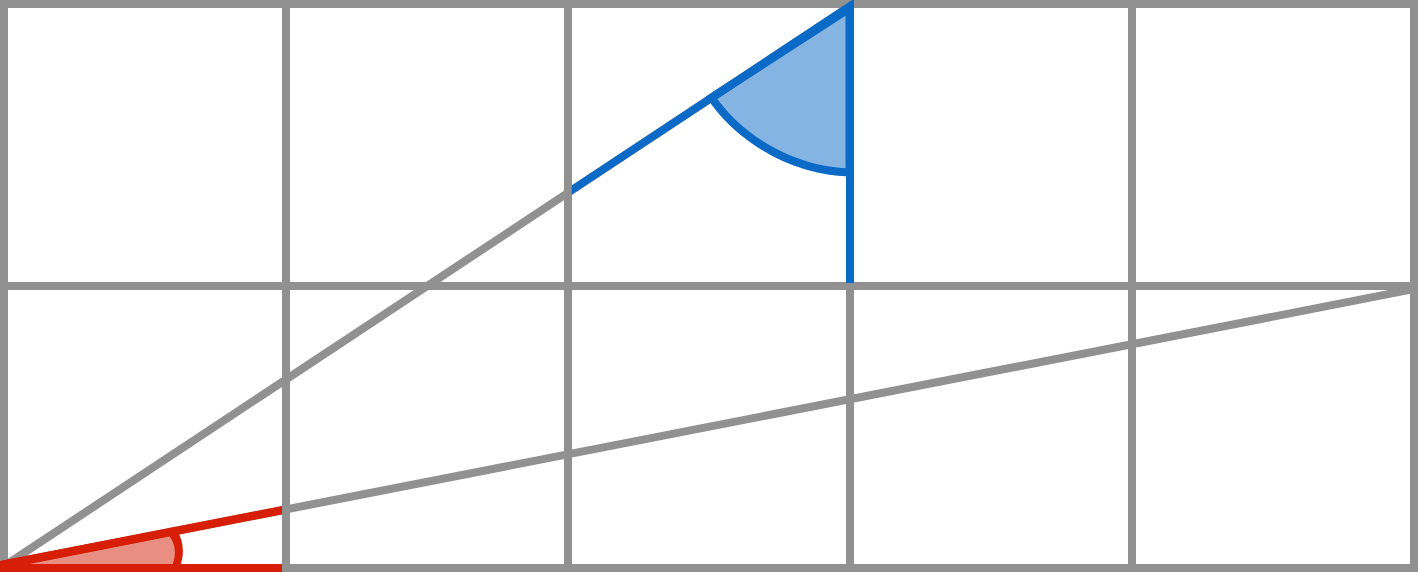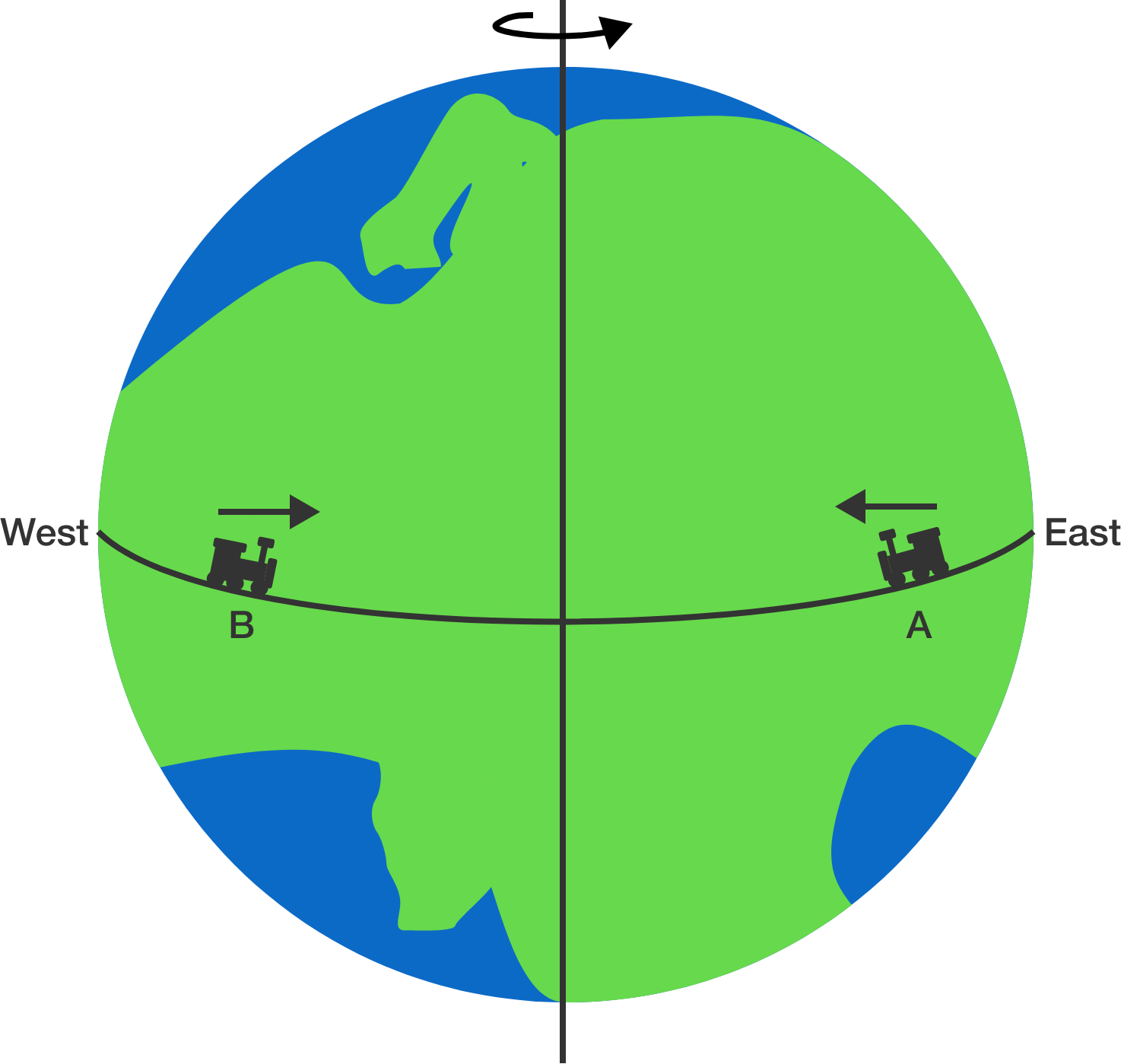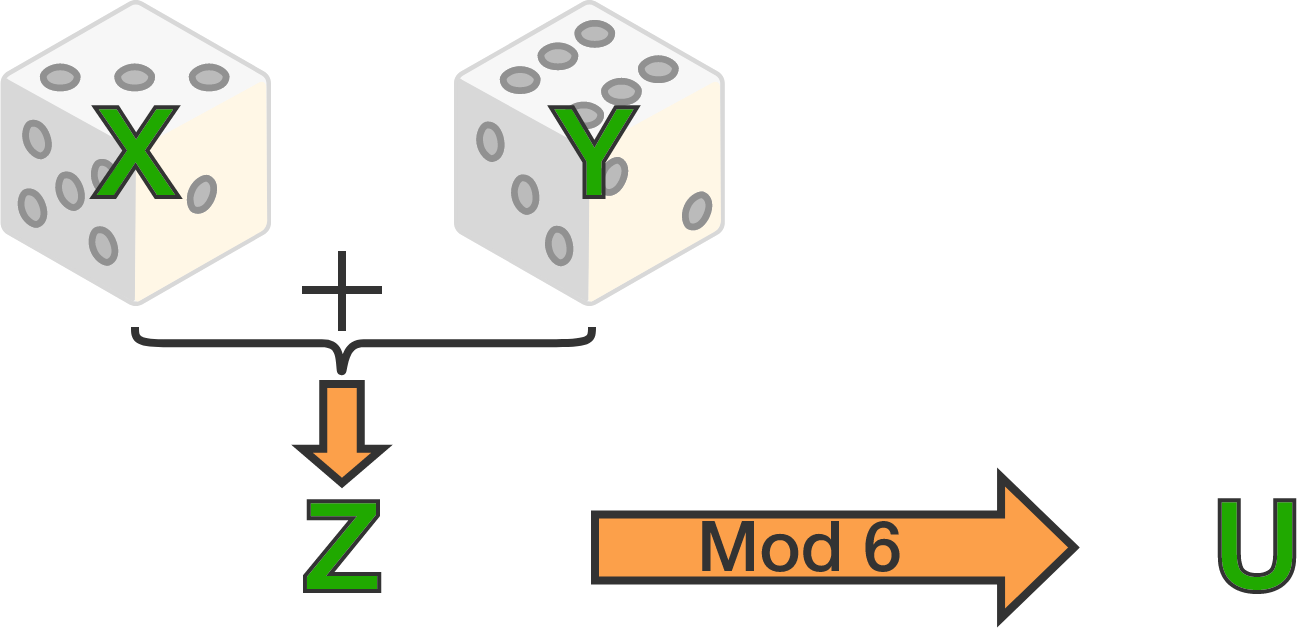# 2017-04-10 IntermediateAs shown by the calculators, the first 3 decimal places of $\sqrt{ 79 } - 8$ and $\sqrt{320} - 17$ are both 0.888.

Which is larger?The rectangle above is divided into 10 squares.

What is ${\color{#3D99F6} \text{(blue angle)}} - {\color{#D61F06} \text{(red angle)}}$ in degrees?Zebedee secretly writes down two consecutive positive integers. Then he tells one of the numbers to Xanthe, and the other number to Yvette (without telling them each other's number, but only telling their numbers are consecutive). The following conversation ensues:

Xanthe: "I don't know your number."
Yvette: "I don't know your number."
Xanthe: "Now I know your number!"
Yvette: "Now I know your number!"

Which of the following must be one of the two numbers?

Note: A randomly chosen correct and complete solution writer will receive a copy of Alex's new puzzle book, Can You Solve My Problems?.Two trains of equal masses are running on a track along the equator of the Earth. Train $A$ runs from east to west, and train $B$ from west to east. The speedometers of the trains show that they are running at equal and constant speeds.

Which train exerts a greater force on the track?A fair die is thrown two times independently. Let $X$ and $Y$ be the random variable indicating the outcomes of these two throws. Define $Z = X + Y,$ and define $U$ as the remainder when $Z$ is divided by $6$.

Which of the following statements are true?

1. $X$ and $Z$ are independent.
2. $X$ and $U$ are independent.
3. $Z$ and $U$ are independent.
×

Problem Loading...

Note Loading...

Set Loading...